# Race Around Condition in JK Flip Flop and T Flip Flop

In this tutorial, we will learn about the issues that occur in JK Flip Flop which is known as race around condition, and how to eliminate this issue. Also, learn about T Flip Flop, its construction, and working. By Saurabh Gupta Last updated : May 11, 2023

## Race Around Condition in JK Flip Flop

Although, JK flip-flop resolves the invalid state condition of SR flip flop, which occurs when Set and Reset are both set to 1. There arises a new problem in JK flip flop, when J and K inputs of the JK flip flop are provided with high input i.e., 1, then output continuously toggles into that region (output changes either from 0 to 1 or from 1 to 0, which creates a disturbance in output. This situation is referred to as the race around the condition.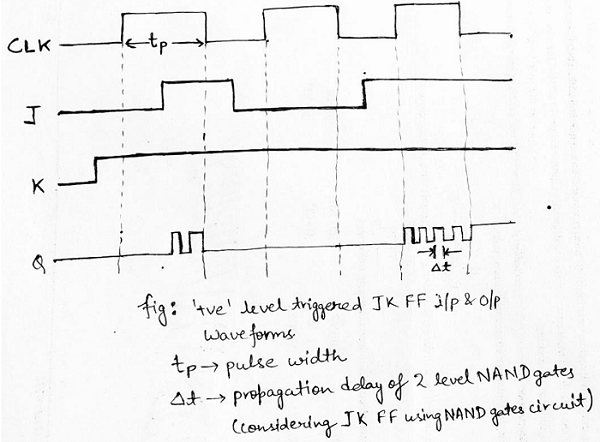## How Can We Eliminate Race Around Condition?

There are three ways using which we can eliminate the race around condition in JK flip flop, which are discussed below:

1. Race around condition exists when tp ≥ Δt. Thus, by keeping tp < Δt, we can avoid race around condition.
2. Use of edge triggering in flip flops.
3. By using a master-slave flip-flop.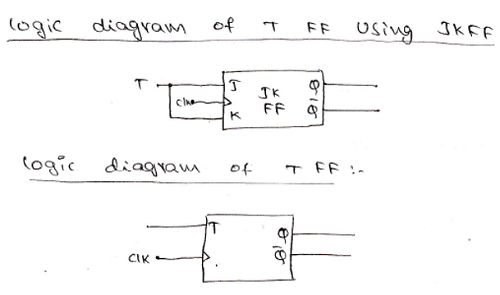## What is T Flip Flop in Digital Electronics?

T Flip Flop is a modification of the JK flip flop. When we join both J and K inputs of the JK-flip flop, then a T Flip Flop is formed. The 'T' in T Flip Flop stands for Toggle. Logic diagram of a positive edge-triggered T Flip Flop is represented as:

### Construction of T Flip Flop

As already discussed, it is formed using joining both the inputs of JK-flip flop to make it a single input T. The logic circuit diagram of T Flip Flop is drawn as: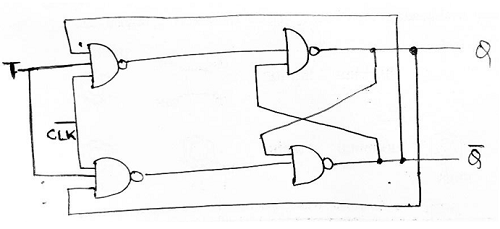From the above given logic circuit, the truth table of the T Flip Flop can be given as: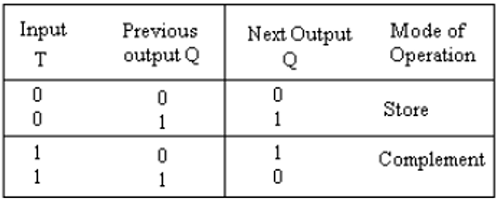### Working of T Flip Flop

T Flip Flop has only two options either has low state (0) or high state (1).

Case 1: When T=0, the flip flop remains in-store mode that means whatever output was obtained in the previous state the same output will be generated in the next state, i.e., there is no change in output.

Case 2: When T=1, the flip flop remains in toggle state/complement mode that means it gives complemented output of the previous state value as the output in the next state.

Input and output waveforms of a positive edge-triggered T Flip Flop can be drawn as: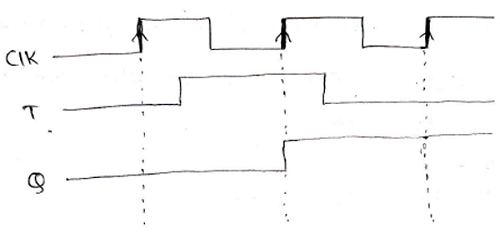Also, from the above given truth table we can derive the characteristic table of T Flip Flop as: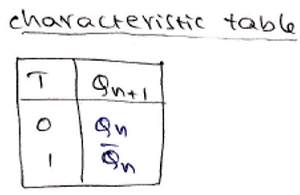Also, the characteristic equation can be derived using the K-Map as: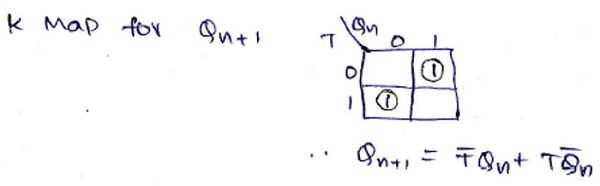Qn+1 = T'Qn + TQn'